Equality axioms

Axioms regularizing the use of the equality relation in mathematical proofs. These axioms assert the reflexivity of the equality relation and the possibility of substituting equals for equals. Symbolically the equality axioms are written: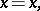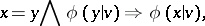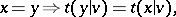whereis a formula andis a term in the language in question,,andare variables having the same non-empty domain of variation, and expressions of the form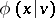and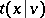denote the result of replacing all free occurrences ofinorby.

Using equality axioms, the symmetry and transitivity of the equality relation can be proved. To do this taketo be the formula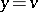in the first case and the formulain the second.

If the formulas and terms of the language in question are constructed from atomic formulas and terms using logical connectives and superposition, then the reduced equality axioms can be derived from their particular cases whenandare atomic formulas and terms. Symbolically: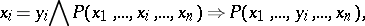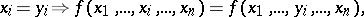whereandare-place predicate and function symbols.